# 1765 On This Memorial Day

### Today’s Puzzle:

This weekend I laid a bouquet of red and white flowers on my husband’s grave and decided to make a red rose Memorial Day puzzle for the blog as well. It is a mystery-level puzzle.

Write the number from 1 to 12 in both the first column and the top row so that those numbers are the factors of the given clues. There is only one solution.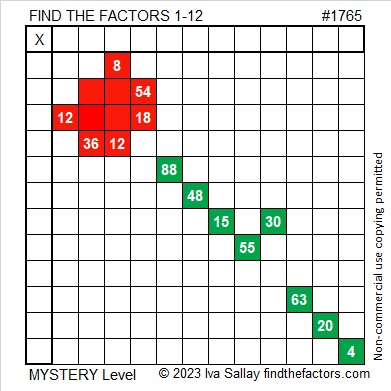### Factors of 1765:

• 1765 is a composite number.
• Prime factorization: 1765 = 5 × 353.
• 1765 has no exponents greater than 1 in its prime factorization, so √1765 cannot be simplified.
• The exponents in the prime factorization are 1 and 1. Adding one to each exponent and multiplying we get (1 + 1)(1 + 1) = 2 × 2 = 4. Therefore 1765 has exactly 4 factors.
• The factors of 1765 are outlined with their factor pair partners in the graphic below.### More About the Number 1765:

1765 is the sum of two squares in two different ways:
42² + 1² = 1765, and
33² + 26² = 1765.

1765 is the hypotenuse of FOUR Pythagorean triples:
84 1763 1765, calculated from 2(42)(1), 42² – 1², 42² + 1²,
413 1716 1765, calculated from 33² – 26², 2(33)(26), 33² + 26²,
1059-1412-1765, which is (3-4-5) times 353, and
1125-1360-1765, which is 5 times (225-272-353).

1765 is a digitally powerful number:
1⁴ + 7³ + 6⁴ + 5³ = 1765.

1765 is a palindrome in a couple of different bases:
It’s A5A base 13 because 10(13²) + 5(13) + 10(1) = 1765, and
it’s 1D1 base 36 because 1(36²) + 13(36) + 1(1) = 1765.

# 1763 Daffodil Puzzle

### Today’s Puzzle:

Spring has sprung and perhaps flowers are blooming in your area. I think my favorite flowers are daffodils. I love the way they are shaped and their vibrant colors.

This daffodil puzzle is a great way to welcome spring. It may be a little bit tricky, but I think if you carefully use logic you will succeed! Just write each of the numbers 1 to 12 in the first column and again in the top row so that those numbers are the factors of the given clues. As always there is only one solution.Here’s the same puzzle if you’d like to print it using less ink:### Factors of 1763:

• 1763 is a composite number and the product of twin primes.
• Prime factorization: 1763 = 41 × 43.
• 1763 has no exponents greater than 1 in its prime factorization, so √1763 cannot be simplified.
• The exponents in the prime factorization are 1 and 1. Adding one to each exponent and multiplying we get (1 + 1)(1 + 1) = 2 × 2 = 4. Therefore 1763 has exactly 4 factors.
• The factors of 1763 are outlined with their factor pair partners in the graphic below.### More About the Number 1763:

1763 is the difference of two squares in two different ways:
882² – 881² = 1763, and
42² – 1² = 1763. (That means the next number will be a perfect square!)

1763 is the hypotenuse of a Pythagorean triple:
387-1720-1763, which is (9-40-41) times 43.

1763 is palindrome 3E3 in base 22 because
3(22²) + 14(22) + 3(1) = 1763.

Lastly and most significantly: 15, 35, 143, 323, 899, and 1763 begin the list of numbers that are the product of twin primes. 1763 is just the sixth number on that list! If we include the products of two consecutive primes whether they are twin primes or not, the list is still fairly small. How rarely does that happen?

When it was 2021, did you realize how significant that year was?

# 1761 Irish Harp

### Today’s Puzzle:

This mystery-level puzzle was meant to look a little like an Irish harp. Using logic write the numbers 1 to 12 in the first column and again in the top row so that those numbers and the given clues make a multiplication table. There is only one solution.Notice that the clues 16 and 24 appear THREE times in the puzzle. In each case, you will need to determine if the common factor is 2, 4, or 8. You will have to get the common factor for each one in the right place or it will cause trouble for another clue. Consider what problems each of the following scenarios bring to other clues. For example, 48 must be either 4 × 12 or 6 × 8, but both possibilities are impossible in at least one of these scenarios: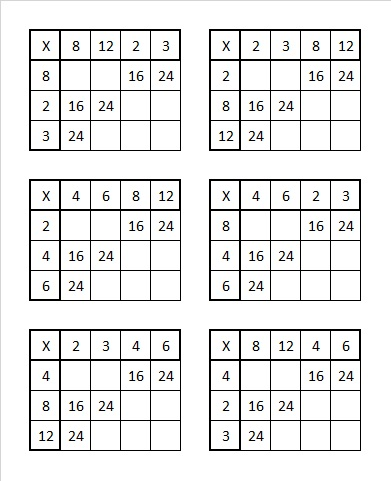Once you determine the only scenario that doesn’t present a problem for any other clue, you will be able to begin the puzzle.

Here’s the same puzzle without any added color:### Factors of 1761:

1 + 7 + 6 + 1 = 15, a number divisible by 3, so 1761 is divisible by 3. Since 6 is divisible by 3, we didn’t have to include it in our sum: 1 + 7 + 1 = 9, so 1761 is divisible by 3.

• 1761 is a composite number.
• Prime factorization: 1761 = 3 × 587.
• 1761 has no exponents greater than 1 in its prime factorization, so √1761 cannot be simplified.
• The exponents in the prime factorization are 1 and 1. Adding one to each exponent and multiplying we get (1 + 1)(1 + 1) = 2 × 2 = 4. Therefore 1761 has exactly 4 factors.
• The factors of 1761 are outlined with their factor pair partners in the graphic below.### More About the Number 1761:

1761 is the difference of two squares in two different ways:
881² – 880² = 1761, and
295² – 292² = 1761.

1761 is palindrome 1N1 in base 32
because 1(32²) + 23(32) + 1(1) = 1761.

# 1760 Pots of Gold and Rainbows

### Today’s Puzzle:

Write the numbers 1 to 12 in the first column and again in the top row so that those numbers are the factors that make the given clues. It’s a level 6, so it won’t be easy. Finding a leprechaun’s pot of gold isn’t easy either. Still, if you can solve this puzzle, then you will have found some real golden nuggets of knowledge.They say there’s a pot of gold at the end of the rainbow. Where’s the rainbow?

### Factors of 1760:

Puzzle number, 1760, has many factors. It makes a very big factor rainbow!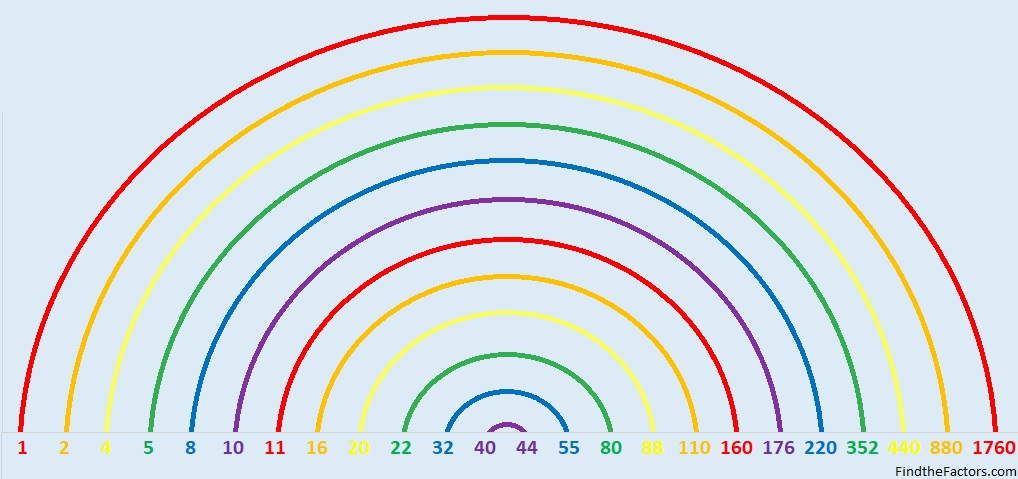• 1760 is a composite number.
• Prime factorization: 1760 = 2 × 2 × 2 × 2 × 2 × 5 × 11, which can be written 1760 = 2⁵ × 5 × 11.
• 1760 has at least one exponent greater than 1 in its prime factorization so √1760 can be simplified. Taking the factor pair from the factor pair table below with the largest square number factor, we get √1760 = (√16)(√110) = 4√110.
• The exponents in the prime factorization are 5, 1, and 1. Adding one to each exponent and multiplying we get (5 + 1)(1 + 1)(1 + 1) = 6 × 2 × 2 = 24. Therefore 1760 has exactly 24 factors.
• The factors of 1760 are outlined with their factor pair partners in the graphic below.### More About the Number 1760:

1760 is the hypotenuse of a Pythagorean triple:
1056-1408-1760 which is (3-4-5) times 352.

1760 is the difference of two squares in eight different ways:
441² – 439² = 1760,
222² – 218² = 1760,
114² – 106² = 1760,
93² – 83² = 1760,
63² – 47² = 1760,
54² – 34² = 1760,
51² – 29² = 1760,  and
42² – 2² = 1760. (That means we are only four numbers away from the next perfect square!)

1760 is palindrome 2102012 in base 3
because 2(3⁶)+1(3⁵)+ 0(3⁴)+2(3³)+0(3²)+1(3¹)+2(3º) = 1760.

# 1759 Pie Over Two

### Today’s Puzzle:

Today in the United States many students will celebrate pi day by eating pie. Today’s puzzle looks a little like a pie that has been cut in half, so I’m calling it pie over two, abbreviated as “π/2”.

Write the numbers 1 to 12 in the first column and again in the top row so that those numbers and the given clues make a multiplication table. Be sure to use logic every step of the way.### Factors of 1759:

• 1759 is a prime number.
• Prime factorization: 1759 is prime.
• 1759 has no exponents greater than 1 in its prime factorization, so √1759 cannot be simplified.
• The exponent in the prime factorization is 1. Adding one to that exponent we get (1 + 1) = 2. Therefore 1759 has exactly 2 factors.
• The factors of 1759 are outlined with their factor pair partners in the graphic below.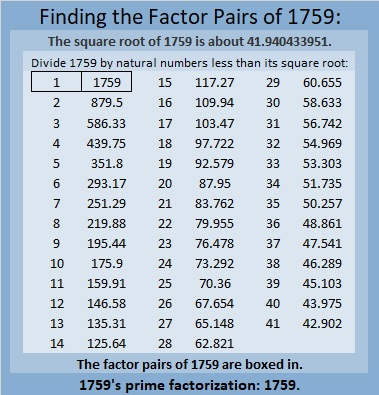How do we know that 1759 is a prime number? If 1759 were not a prime number, then it would be divisible by at least one prime number less than or equal to √1759. Since 1759 cannot be divided evenly by 2, 3, 5, 7, 11, 13, 17, 19, 23, 29, 31, 37, or 41, we know that 1759 is a prime number.

### More About the Number 1759:

Like every other odd number, 1759 is the difference of two squares:
880² – 879² = 1759.

OEIS.org informs us that 1759 is only the 17th Eisenstein-Mersenne prime number.

# 1758 Two-Shillelagh O’Sullivan

### Today’s Puzzle:

When I was looking for the song about the shillelagh for my previous post, I found another one called Two-Shillelagh O’Sullivan also by Bing Crosby. It wasn’t a song from my childhood, but it inspired me to make a puzzle with two shillelaghs anyway. In the song, O’Sullivan wears these walking sticks in a holster and can draw them quicker than anyone can draw a gun. He was impossible to beat.

This two-shillelagh puzzle is also a bit difficult to beat. You’re not going to let that stop you from trying, are you? Just use logic and your knowledge of the multiplication table.

Write the numbers from 1 to 12 in the first column and again in the top row so that those numbers are the factors of the given clues.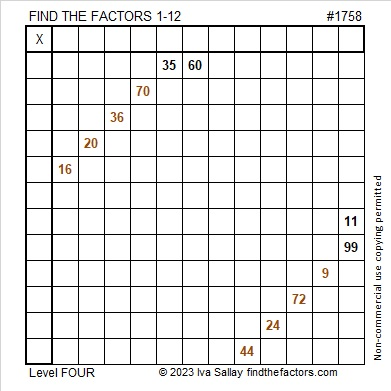Here’s the same puzzle in black and white: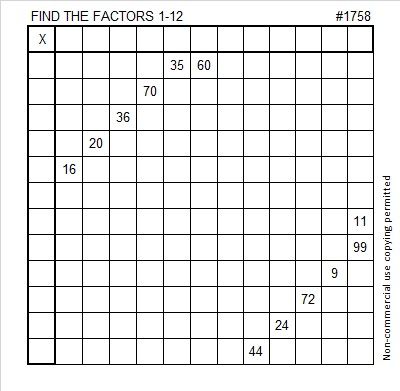### Factors of 1758:

• 1758 is a composite number.
• Prime factorization: 1758 = 2 × 3 × 293.
• 1758 has no exponents greater than 1 in its prime factorization, so √1758 cannot be simplified.
• The exponents in the prime factorization are 1, 1, and 1. Adding one to each exponent and multiplying we get (1 + 1)(1 + 1)(1 + 1) = 2 × 2 × 2 = 8. Therefore 1758 has exactly 8 factors.
• The factors of 1758 are outlined with their factor pair partners in the graphic below.### More About the Number 1758:

1758 is the hypotenuse of a Pythagorean triple:
408-1710-1758, which is 6 times (68-285-293).

1758 is palindrome 8E8 in base 14
because 8(14²) + 13(14) + 8(1) = 1758.

# 1757 A Shillelagh Puzzle

### Today’s Puzzle:

When I was in elementary school, one of my teachers had us listen to a variety of songs for Saint Patrick’s Day including Bing Crosby’s It’s the Same Old Shillelagh. It’s almost Saint Patrick’s Day, so here’s a puzzle that looks a little like a shillelagh, an Irish walking stick.

Since this is a level-3 puzzle, begin with the clues at the top of the puzzle and work your way down using each number 1 to 12 once in the first column and once in the top row. The numbers you write and the given clues need to form a multiplication table. There is only one solution.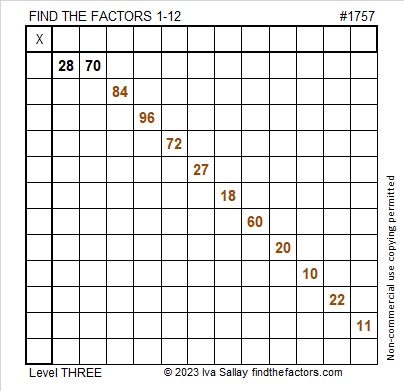Here is the same puzzle in black and white:### Factors of 1757:

• 1757 is a composite number.
• Prime factorization: 1757 = 7 × 251.
• 1757 has no exponents greater than 1 in its prime factorization, so √1757 cannot be simplified.
• The exponents in the prime factorization are 1 and 1. Adding one to each exponent and multiplying we get (1 + 1)(1 + 1) = 2 × 2 = 4. Therefore 1757 has exactly 4 factors.
• The factors of 1757 are outlined with their factor pair partners in the graphic below.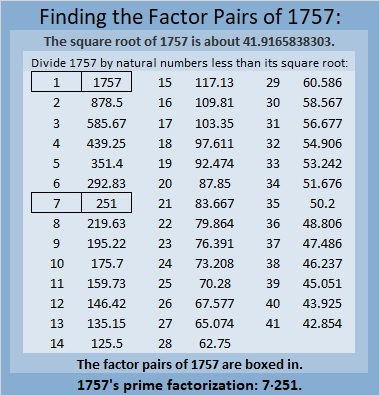### More About the Number 1757:

1757 is the difference of two squares in two different ways:
879² – 878² = 1757, and
129² – 122² = 1757.

1757 is palindrome 616 in base17
because 6(17²) + 1(17) + 6(1) = 1757.

# When Does 1756 Look a Little Like π?

### Today’s Puzzle:

Here are 1756 tiny squares. Solve for n and you will know which centered pentagonal number 1756 is. You could also count the pentagons from the center outward.What triangular number multiplied by 5 is one less than 1756?

How do you pronounce pentagonal? Here’s a quick video on its correct pronunciation:

Confession: I had been mispronouncing all of those terms, but not anymore!

### Factors of 1756:

• 1756 is a composite number.
• Prime factorization: 1756 = 2 × 2 × 439, which can be written 1756 = 2² × 439.
• 1756 has at least one exponent greater than 1 in its prime factorization so √1756 can be simplified. Taking the factor pair from the factor pair table below with the largest square number factor, we get √1756 = (√4)(√439) = 2√439.
• The exponents in the prime factorization are 2 and 1. Adding one to each exponent and multiplying we get (2 + 1)(1 + 1) = 3 × 2 = 6. Therefore 1756 has exactly 6 factors.
• The factors of 1756 are outlined with their factor pair partners in the graphic below.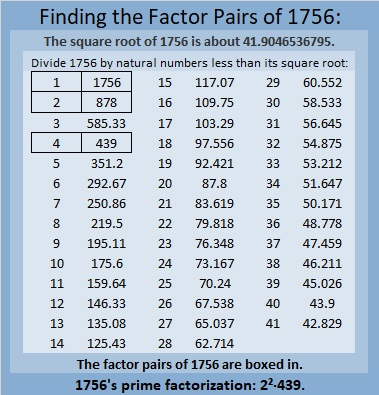### More About the Number 1756:

The centered pentagon above doesn’t really look like π, so when does 1756 look a little bit like π? Pi day in America is next week, so this is a time-sensitive question.

Actually,1756 looks a little like 10π in base 24. You see 1756₁₀ is the same as 314₂₄.
Why? Because 3(24²) + 1(24) + 4(1) = 1756.

1756₁₀ also is the same as 1024₁₂.
Why? Because 1(12³) + 0(12²) + 2(12) + 4(1) = 1756.
I think that’s cool because 2¹º = 1024.

1756 is the difference of two squares:
440² – 438² = 1456.

# 1754 and a Level 2 Puzzle

### Today’s Puzzle:

Can you write each number from 1 to 12 in the first column and again in the top row so that the products of the numbers you write are the given clues?### Factors of 1754:

• 1754 is a composite number.
• Prime factorization: 1754 = 2 × 877.
• 1754 has no exponents greater than 1 in its prime factorization, so √1754 cannot be simplified.
• The exponents in the prime factorization are 1 and 1. Adding one to each exponent and multiplying we get (1 + 1)(1 + 1) = 2 × 2 = 4. Therefore 1754 has exactly 4 factors.
• The factors of 1754 are outlined with their factor pair partners in the graphic below.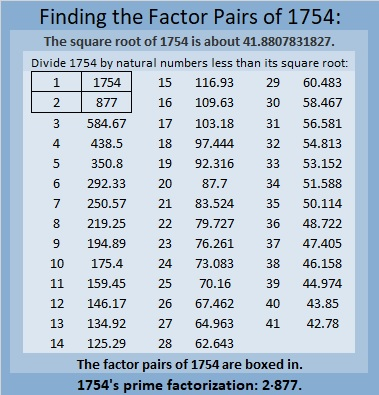### More About the Number 1754:

1754 is the sum of two squares:
35² + 23² = 1754.

1754 is the hypotenuse of a Pythagorean triple:
696-1610-1754, calculated from 35² – 23², 2(35)(23), 35² + 23².
It is also 2 times (348-805-877).

# 1752 So Many Snowflakes

### Today’s Puzzle:

A winter storm hit much of the United States today including the drought-stricken area where I live. We are so grateful for the snow, but also grateful we could stay home all day. Driving in this much snow is not much fun.

This snowflake mystery-level puzzle might have a couple of tricky clues, but I’m confident that you’ll still find it easier than clearing a couple of feet of snow off a driveway! Just write the numbers 1 to 12 in both the first column and the top row so that those numbers and the given clues make a multiplication table.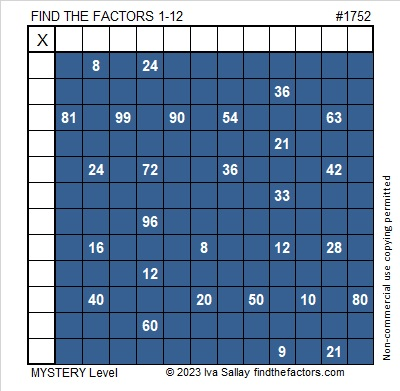Here’s the same puzzle without any added color: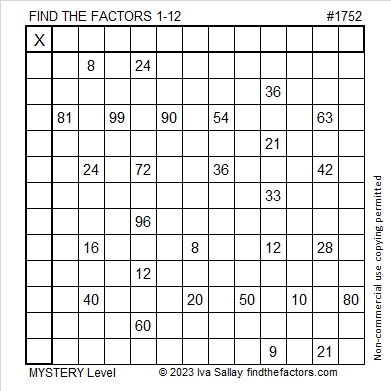### Factors of 1752:

• 1752 is a composite number.
• Prime factorization: 1752 = 2 × 2 × 2 × 3 × 73, which can be written 1752 = 2³ × 3 × 73.
• 1752 has at least one exponent greater than 1 in its prime factorization so √1752 can be simplified. Taking the factor pair from the factor pair table below with the largest square number factor, we get √1752 = (√4)(√438) = 2√438.
• The exponents in the prime factorization are 3,1 and 1. Adding one to each exponent and multiplying we get (3 + 1)(1 + 1) (1 + 1) = 4 × 2 × 2 = 16. Therefore 1752 has exactly 16 factors.
• The factors of 1752 are outlined with their factor pair partners in the graphic below.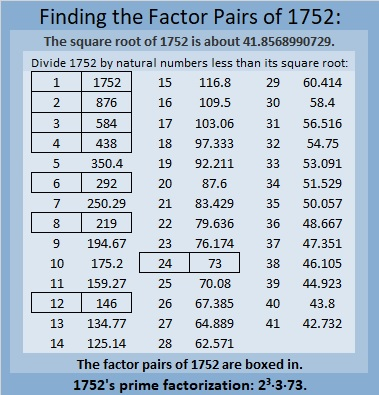### More About the Number 1752:

1752 is the hypotenuse of a Pythagorean triple:
1152 1320 1752, which is 24 times (48-55-73).

1752 is the difference of two squares in FOUR different ways:
439² – 437² = 1752,
221² – 217² = 1752,
149² -143² = 1752, and
79² – 67² = 1752.

1752 is a palindrome in some other bases:
It’s A4A in base13 because 10(13²) + 4(13) + 10(1) = 1752,
it’s 4G4 in base19 because 4(19²) + 16(19) + 4(1) = 1752, and
it’s 2K2 in base 25 because 2(25²) + 20(25) + 2(1) = 1752.